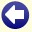## Solution to: Weighing Marbles

Number the ten bags from 1 up to and including 10. Take the following number of marbles from each bag:

 Bag number Number of marbles 1 1 2 2 3 4 4 8 5 16 6 32 7 64 8 128 9 256 10 512

As you can see, the numbers of marbles are powers of 2.

Place all 1023 marbles that you took from the bags together on the balance. From the number of grams that the total weight of these marbles differs from 10230 grams, you can deduce which bags (if any) contain the marbles of 9 grams. This is done by looking which of the powers of 2, as indicated in the table above, make up the difference.

For example, if the weight of the marbles is 9900 grams, the difference is 10230 grams - 9900 grams = 330 grams. Then look which powers of 2, from highest to lowest, can successively be subtracted from 330. In this way, it can be found that 330 = 256 + 64 + 8 + 2. Hence, bags 9, 7, 4, and 2 contain the marbles of 9 grams in this example.Back to the puzzle
This website uses cookies. By further use of this website, or by clicking on 'Continue', you give permission for the use of cookies. If you want more information, look at our cookie policy.